# Class 9 Maths Worksheet for Probability

Given below are the Class 9 Maths Assignments for Probability
a. Concepts questions
b. Calculation problems
c. Multiple choice questions
d. Long answer questions
e. Fill in the blank's
f. Link type comprehension
Question 1.
1000 families with 2 children were selected randomly and the following data were recorded: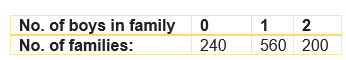If a family is chosen at random, find the probability that it has
1. No boy
2. One boy
3. 2 boys
4. at least one boy
5. at most 1 boy
Total Number of Families =$240 + 560 + 200= 1000$
a. P( No boy) = $\frac {240}{1000} = \frac {6}{25}$
b. P( one boy) = $\frac {560}{1000} = \frac {14}{25}$
c. P( twp boy) = $\frac {200}{1000} = \frac {1}{5}$
d. P( at least one boy) = $\frac {200 + 560}{1000} = \frac {19}{25}$
d. P( at most 1 boy) = $\frac {240 + 560}{1000} = \frac {4}{5}$

Question 2.
The percentage of marks obtained by a student in the monthly unit tests are given below: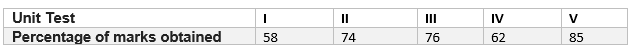Find the probability that the students gets:
1. a first class i.e. at least 60% marks
2. marks between 70% and 80%
3. a distinction i.e. 75% or above
4. less than 65% marks
Total Number of students =$58 + 74 + 76 + 62 + 85= 355$
a. P( a first class i.e. at least 60% marks) = $\frac {62 + 74 + 76 + 85}{355} = \frac {297}{355}$
b. P(marks between 70% and 80%) = $\frac {74 + 76}{355} = \frac {30}{71}$
c. P(a distinction i.e. 75% or above) = $\frac {76 + 85}{355} = \frac {161}{355}$
d. P(less than 65% marks) = $\frac {62+ 58}{355} = \frac {24}{71}$

Question 3.
On the page of a telephone directory, there were 200 telephone numbers. The frequency distribution of their unit place digit(for example, in the number 55828573, the unit place is 3) is given in the table below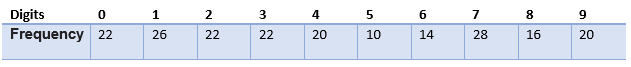A no. is chosen at random, find the probability that the digit at the unit’s place is:
1. 9
2. a non- zero multiple of 3
3. a non- zero even no.
4. an odd no.
Total Number of telephone numbers =200
a. P( 9) = $\frac {20}{200} = \frac {1}{10}$
b. P(a non- zero multiple of 3) = $\frac {22 + 14+ 20}{200} = \frac {7}{25}$
c. P(a non- zero even no) = $\frac {22 + 20 + 14 + 16}{200} = \frac {9}{25}$
d. P(odd no) = $\frac {26+ 22+ 10+ 28 +20}{200} = \frac {53}{100}$

Question 4.
A tyre manufacturing company kept a record of the distance covered before a tyre to be replaced. Following table shows the results of 1000 cases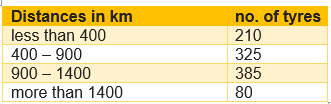If you buy a tyre of this company, what is the probability that:
1. it will need to be replaced before it has covered 400km?
2. it will last more than 900 km?
3. it will need to be replaced after it has covered somewhere between 400km and 1400km?
4. it will not need to be replaced at all?
5. it will need to be replaced?
Total Number of Tyres =1000
a. P( it will need to be replaced before it has covered 400km) = $\frac {210}{1000} = \frac {21}{100}$
b. P(it will last more than 900 km) = $\frac {385 + 80}{1000} = \frac {93}{200}$
c. P(it will need to be replaced after it has covered somewhere between 400km an 1400km?) = $\frac {325 + 385 + 80 }{1000} = \frac {79}{100}$
d. P(it will not need to be replaced at all) = $\frac {0}{1000} = 0$
d. P(it will need to be replaced) = $\frac {1000}{1000} = 1$

Question 5.
Fifty seeds were selected at random from each of 5 bags of seeds, and were kept under standardized conditions favorable to germination. After 20 days the number of seeds which had germinated in each collection were counted and recorded as follows-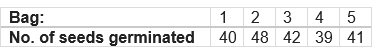What is the probability of germination of:
1. more than 40 seeds in a bag?
2. 49 seeds in a bag?
3. More than 35 seeds in a bag?
4. at least 40 seeds in a bag?
5. at most 40 seeds in a bag?
Total Number of Bagss=5
a. P( more than 40 seeds in a bag) = $\frac {4}{5}$
b. P(49 seeds in a bag) = $\frac {0}{5} = 0$
c. P(More than 35 seeds in a bag) = $\frac {5 }{5} = 1$
d. P(at least 40 seeds in a bag) = $\frac {5 }{5} = 1$
d. P(at most 40 seeds in a bag) = $\frac {1}{5}$

Question 6.
The distances (in km) of 40 female engineers from the residence to their place of work were found as follows: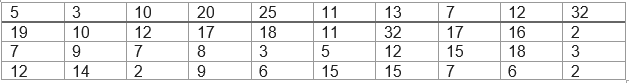Find the probability that the engineer lives:
1. less than 7km from her place of work?
2. at least 7km from her place of work?
3. Within 1/2km from her place of work?
4. at most 15km from her place of work?
Total Number of Bagss=5
a. P( less than 7km from her place of work) = $\frac {10}{40}= \frac {1}{4}$
b. P(at least 7km from her place of work) = $\frac {30}{40} = \frac {3}{4}$

c. P(Within 1/2km from her place of work) = $\frac {0 }{40} = 0$
d. P(at most 15km from her place of work) = $\frac {29 }{40}$

Question 7.
Following table shows the marks scored by a group of 90 students in a mathematics test of 100 marks.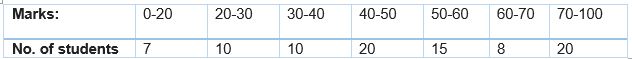Find the probability that the student obtains
a. less than 20
b. 60 or more
Total Number of Bagss=5
a. P( less than 20) = $\frac {7}{90}$
b. P(60 or more) = $\frac {8 + 20}{90} = \frac {14}{45}$

Question 8.
Following frequency distribution gives the weight of 38 students of a class: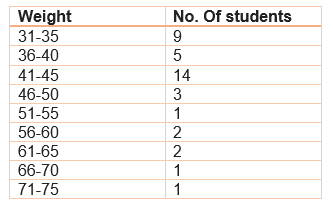Find the probability that weight of the students in the class is:
1. at most 60kg
2. at least 36kg
3. not more than 50kg
4. Also define two events in this context, one having probability 0 and other having 1.

Question 9.
An insurance company selected 2000 drivers at random in a particular city to find a relationship return age and accidents. The data obtained are given in the following table: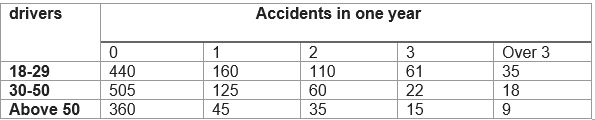Find the probabilities of following events for a driver chosen at random from the life city:
1. being 18-29 year of age and having exactly 3 accidents in one year.
2. being 30-50 years of age and having one or more accidents in a year.
3. having no accidents in one year

Question 10.
A recent survey found that the ages fo workers in a factory is distributed as follows: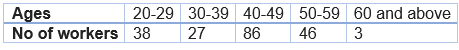if a person is selected at randomly, find the probability that a person is
a. 40 yrs or more
b. under 40 yrs
c. having age from 30 to 39 yrs
d. under 60 but over 39

Question 11.
The blood groups of 30 students of class IX are recorded as follows:
A, B, O, O, AB, O, A, O, A, O, B, A, O, O, A, AB, O, A, A, O, O, AB, B, A, O, B, A, B, O.
A student is selected at random from the class for blood donation. Find the probability that the blood group of student chosen is
a. A b. B
c. AB
d. O

Question 12.
A company selected 2400 families at random and surveys them to determine a relationship between income level and the no. of vehicles in a home. The information gathered is listed in the table below: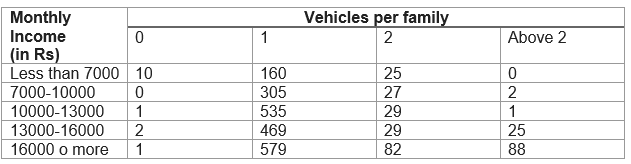If a family is chosen at random, find the probability that the family is-
1. earning Rs 10000-13000 month and owing exactly 2 vehicles.
2. earning Rs 16000 or more per month and owing exactly 1 vehicle.
3. earning less than R 7000 per month and does not own any vehicle.
4. earning Rs 13000-16000 month and owing more than 2 vehicles.
5. owning not more than 1 vehicle
6. owning at least one vehicle.

Question 13.
The following table gives the life time of 400 neon lamps: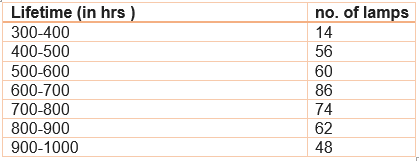A bulb is selected at random. Find the probability that the life time of selected bulb is:
1. less than 400
2. between 300-800hrs
3. at least 700 hrs

Question 14.
Given below is the frequency distribution of wages (in Rs) of 30 workers in a certain factory: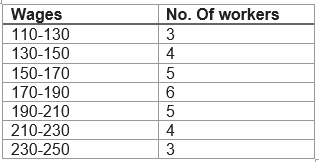A worker is selected at random. Find the probability that his wages are:
1. less than Rs 150
2. at least Rs 210
3. more than or equal to 150 but less than Rs 210.

Question 15.
If the probability of winning a Hockey match is one half more than twice of probability of losing the match, find the probabilities of
1. winning of the match
2. losing of the match
Answer: Let x be the probability of losing a Hockey match
Then probability of winning a Hockey match = $2x + \frac {1}{2}$
Now $x + 2x + \frac {1}{2} =1$
$3x = \frac {1}{2}$
$x = \frac {1}{6}$

Now of Winning = $2x + \frac {1}{2}= \frac {1}{3} + \frac {1}{3} = \frac {5}{6}$

Question 16.
The probability of guessing the correct answer to a certain questions is $\frac {x}{12}$. If the probability of not guessing the correct answer is $\frac {3}{4}$. then find the value of x.
P(Correct)=$\frac {x}{12}$.
P(Not coreect)=$\frac {3}{4}$
P(Not Correct) + P(Correct) + 1
$\frac {3}{4} + \frac {x}{12}=1$
$x = 3$

Question 17.
If the difference between the probability of success and failure of an event is $\frac {5}{19}$, find the probabilities of success and failure of an event.
Let the probability of success = a
Let the probability of failure = b
so, $a + b = 1$
Also given , $x - y = \frac {5}{19}$
Adding both above equation
$2a = 1 + \frac {5}{19}$
$a = \frac {12}{19}$
Now for failure
$\frac {12}{19} + b = 1$
or
$b = =frac {7}{19}$

Question 19.
If the probability of winning a race of an athlete is 1/6 less than twice the probability of losing the race, find the probability of winning the race.
Let the probability of not winning the race be a
Ley probability of winning the race be b

as according to question
$a=2b- \frac {1}{6}$
or
$a -2b =- \frac {1}{6}$ --(1)
Also
$a + b=1$ -(2)
Substracting equation 1 and 2
$b= \frac {7}{18}$
Now From $a + b=$, we get
$a= \frac {11}{18}$

Question 20.
The following table shows the daily production of TV sets in a industry for 7 days of a week.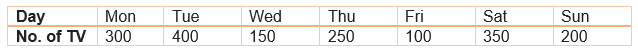If a TV is selected at random, find the probability that it is selected-
1. from a day having maximum production
2. from a day having minimum production
3. from Saturday’s production

Question 22.
A parent has collected data of number of school based on the monthly fees, so that he can choose the school for admission of his child. Data is as under: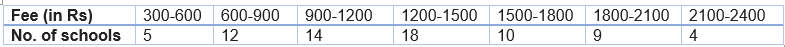If a school is selected at random, find the probability that the school is having –
1. minimum fees
2. maximum fees
3. fees less than 2900
4. fees at least Rs 1500

Question 23.
A survey of 2000 people of different age groups was conduct to find out their preference n watching different types of movies.
Type I – family
Type II – comedy & family
Type III – romantic, comedy & family
Type IV – action, romantic, comedy & family
The data recorded was as follows –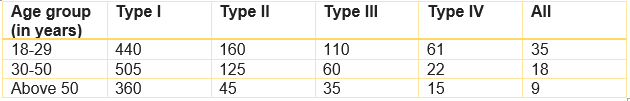Find the probability that person chosen at random is-
1. in 18-29 years of age and likes type II movies
2. above 50 years of age and likes all types of movies
3. in 30-50 years and likes type I movies.

Question 24.
An insurance company selected 2000 drivers at random in a particular city to find a relationship between age and accidents. The data obtained are given in following table-
Age groups of               no. of accidents
Drivers (in years)            0          1          2           3          more than 3
18-29                            440       160      110        61            35
30-50                            505       125        60        21           18
above 50                       360         45        35        15           10
Find the probability:-
1. being 18-29 years of age and having exactly 3 accidents.
2. being 30-50 years of age and having one or more accidents in a year.
3. having no accidents in 1 year.
4. which value would you like to remember from data?

Question 25.
Find the probability that a leap year, selected at random will have 53 Sundays
A normal year(365 days) has 52 Mondays, 52 Tuesdays, 52 Wednesdays, 52 Thursdays, 52 Fridays, 52 Saturdays and 52 Sundays + 1 day( 7 X 52 +1) that could be anything depending upon the year under consideration. In addition to this, a leap year(366) has an extra day which might be a Monday or Tuesday or Wednesday...or Sunday.

So Now our question is reduced to finding the consecutive pairs of the year where one of them is a Sunday

Our sample space is S : {Monday-Tuesday, Tuesday-Wednesday, Wednesday-Thursday,..., Sunday-Monday}
Number of elements in S = n(S) = 7
Now Saturday-Sunday and Sunday-Monday satisfies our requirement(2)
probability of occurrence of 53 Sundays in leap = $\frac {2}{7}$

Question 26.
A coin is tossed 15 times and observed that 11 times head comes up. Find the probability that a tail comes up
No. of heads = 11
no. of tails = 15-11
probability of tails = $\frac {4}{15}$

• Notes
• NCERT Solutions & Assignments